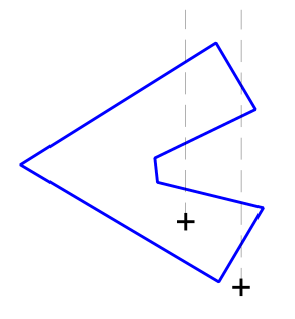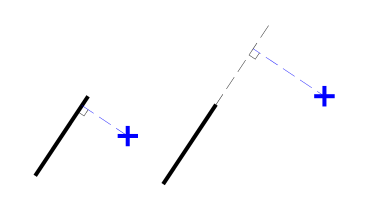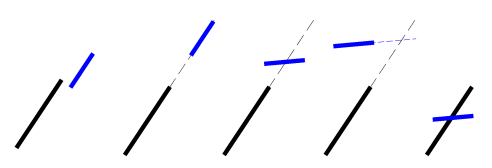## Test if a Point lies inside a Polygon (from CGA FAQ)

The CGA FAQ describes an algorithm to test if a point lies within a polygon. Roughly speaking, you start from the point and travel north. If you cross an odd number of sides, it means that you were within the polygon when you started and vice versa.The details of the solution can be found in the CGA FAQ linked above. A C++ implementation can be found here: http://code.google.com/p/curve-project/source/browse/trunk/lab/cgafaq/cgafaq.h The function cgafaq::point_in_polygon takes a point and a polygon and returns whether or not the point lies inside the polygon. This is a direct translation of the code from the FAQ.

Disclaimer: This post and the code provided are not endorsed by any of the contributors to the CGA FAQ.

## Orientation of Polygons (from CGA FAQ)

The orientation of a polygon tells us if the vertices are ordered clockwise or counter-clockwise as we move along the perimeter. This is determined by calculating the polygon’s signed area which is positive for counter-clockwise orientation and negative for clockwise orientation. A quicker algorithm is to determine the signed area of the triangle formed by the lowest right most vertex and it’s immediate neighbors.

The details of the solution can be found in the CGA FAQ linked above. A C++ implementation can be found here: http://code.google.com/p/curve-project/source/browse/trunk/lab/cgafaq/cgafaq.h The function cgafaq::orientation has two overloads. The first computes orientation for three vertices and the second calculates the orientation of a polygon.

Disclaimer: This post and the code provided are not endorsed by any of the contributors to the CGA FAQ.

## Distance of a Point from a Line (from CGA FAQ)

The distance of a point from a line is the length of a perpendicular drawn from the point to the line. Here are the possibilities:

• Perpendicular meets on the line segment
• Perpendicular meets on a forward/backward extension of the line segmentThe details of the solution can be found in the CGA FAQ linked above. A C++ implementation can be found here: http://code.google.com/p/curve-project/source/browse/trunk/lab/cgafaq/cgafaq.h The function cgafaq::distance_from_line takes the start and end points of the line and the point from which distance is to be calculated. r and s are output parameters calculated as described in the FAQ.

Note that if distance is not important and only a perpendicular needs to be generated then there is a simpler way to construct one.

Disclaimer: This post and the code provided are not endorsed by any of the contributors to the CGA FAQ.

## Intersection of Line Segments (from CGA FAQ)

Intersection of two line segments
involves the following cases:

• Segments are parallel
• Segments are collinear/coincident
• One segment intersects the extension of the other
• Extension of one segment intersects the extension of the other
• Segments intersect each other.The details of the solution can be found in the CGA FAQ linked above. A C++ implementation can be found here: http://code.google.com/p/curve-project/source/browse/trunk/lab/cgafaq/cgafaq.h The function cgafaq::intersect takes 4 points — the start and end points of each of the lines. r and s are output parameters calculated as described in the FAQ.

Disclaimer: This post and the code provided are not endorsed by any of the contributors to the CGA FAQ.

## Perpendiculars

I often need to construct perpendiculars in my code and found a very elegant solution to this problem in CGAL source.

Given points A(xa, ya) and B(xb, yb), perpendiculars BBccw and BBcw to the line AB can be constructed at B using either of the following points:

Bccw(xb – (yb – ya), yb + (xb – xa)) // such that ABBccw is oriented counter-clockwise

or

Bcw(xb + (yb – ya), yb – (xb – xa)) // such that ABBcw is clockwise.

This follows from the fact that the product of the slopes of perpendicular lines is -1.

Here is code to return either of these points.

Output: